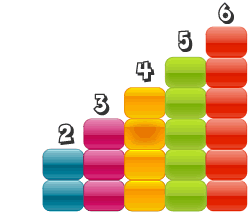# Regret using calculator

Algebra Level 3$\large \color{#20A900}{A=2^{1465}} , \ \ \ \ \ \color{#3D99F6}{B=3^{1172}} , \ \ \ \ \ \color{#D61F06}{C=5^{879}}$

If these numbers are arranged from smallest to biggest in ascending order, what would the order of arrangement be?

×